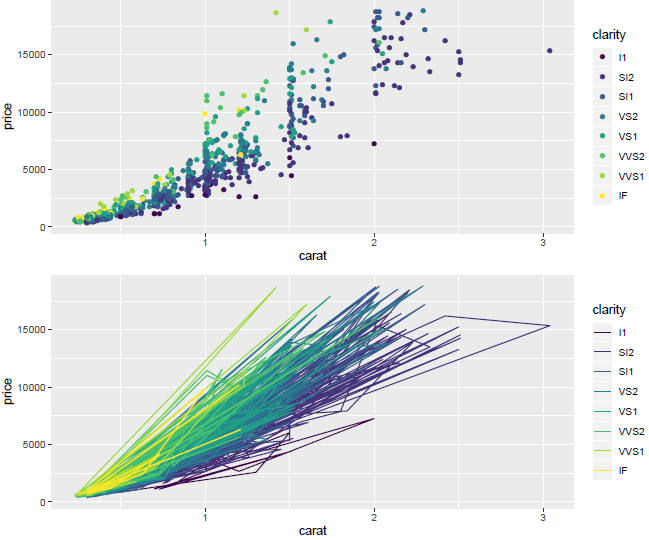2 views

I am trying to plot multiple plots using ggplot2, arranging them using grid.arrange(). Since I managed to find someone describing the exact problem I have, I have quoted from the problem description from the link:

When I use ggsave() after grid.arrange(), i.e.

grid.arrange(sgcir1,sgcir2,sgcir3,ncol=2,nrow=2)

ggsave("sgcirNIR.jpg")

I do not save the grid plot but the last individual ggplot. Is there any way of actually saving the plot as displayed by grid.arrange() using ggsave() or something similar? Other than using the older way

jpeg("sgcirNIR.jpg")

grid.arrange(sgcir1,sgcir2,sgcir3,ncol=2,nrow=2)

dev.off()

The same link gives the solution below:

require(grid)

require(gridExtra)

p <- arrangeGrob(qplot(1,1), textGrob("test"))

grid.draw(p) # interactive device

ggsave("saving.pdf", p) # need to specify what to save explicitly

However, I can't figure out how to use ggsave() to save the output of the grid.arrange() call in the following code:

library(ggplot2)

library(gridExtra)

dsamp <- diamonds[sample(nrow(diamonds), 1000), ]

p1 <- qplot(carat, price, data=dsamp, colour=clarity)

p2 <- qplot(carat, price, data=dsamp, colour=clarity, geom="path")

g_legend<-function(a.gplot){

tmp <- ggplot_gtable(ggplot_build(a.gplot))

leg <- which(sapply(tmp\$grobs, function(x) x\$name) == "guide-box")

legend <- tmp\$grobs[[leg]]

return(legend)}

legend <- g_legend(p1)

lwidth <- sum(legend\$width)

## using grid.arrange for convenience

## could also manually push viewports

grid.arrange(arrangeGrob(p1 + theme(legend.position="none"),

p2 + theme(legend.position="none"),

main ="this is a title",

left = "This is my global Y-axis title"), legend,

widths=unit.c(unit(1, "npc") - lwidth, lwidth), nrow=1)

# What code to put here to save output of grid.arrange()?

by

You can use arrangeGrob function that returns a grob g that you can pass to the ggsave function to save the plot.

Whereas grid.arrange draws directly on a device and by default, the last plot is saved if not specified i.e., the ggplot2 invisibly keeps track of the latest plot.

For example:

library(ggplot2)

require(grid)

library(gridExtra)

dsamp <- diamonds[sample(nrow(diamonds), 1000), ]

p1 <- qplot(carat, price, data=dsamp, colour=clarity)

p2 <- qplot(carat, price, data=dsamp, colour=clarity, geom="path")

g <- arrangeGrob(p1, p2, nrow=3)

ggsave(file="name.pdf", g,width = 8, height = 10)

Output: